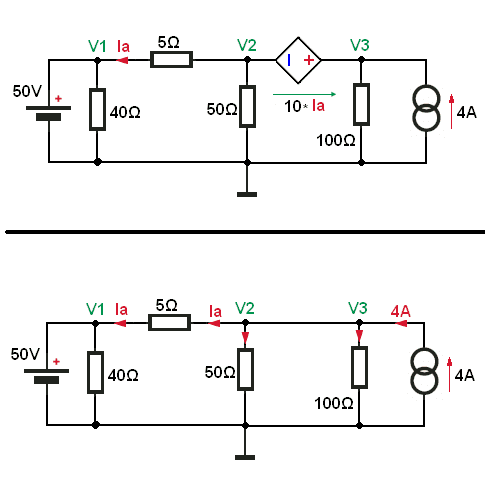Analyzing a circuit with a current dependent voltage source

• Engineering
Hey guys,

I'm trying the analyze a circuit, I have the answers I should be getting but they're way off what I'm getting when I'm trying to work it out.

I think I might be approaching it from the wrong angle, does anyone have any suggestions as to where I might start.

I'm trying to find v3 and v2 (the 2nd and 3rd nodes).

At the moment I'm doing the KCL for each node, and getting three equations with all the node voltages as well as the currents through the voltage source and the current dependant voltage source as variables.

I then do the mesh analysis which I substitute into the original equations, which has given me some pretty crazy answers. The latest being:
v3=696V

v3 is supposed to be 80V!!!

It's driving me insane, and the way I'm doing it means it takes me an hour to redo it everytime.

Any help would be much appreciated.

Attachments

•Circuit1-4.jpg
36.1 KB · Views: 415

Thanks! I thought it would have something to do with a supernode but we've only done them in parallel, I wasn't sure it would still apply.

Also in my last three tries I let v1= 50V, but this time I didn't. Clearly time to head to bed lol.

Thanks again.

Look at my solution.
We have a one supernode, and this means that we treat V2 and V3 as a one supernode.
We short CCVS and use nodal analysis.Now we write KCL for our supernode, 4A current source entering node and remaining currents leaving the node.

4A = V3/100Ω + V2/50Ω + (V2 - 50V)/5Ω (1)